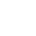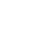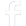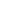#### WhatsApp Quick Chat#### Get Instant Call Back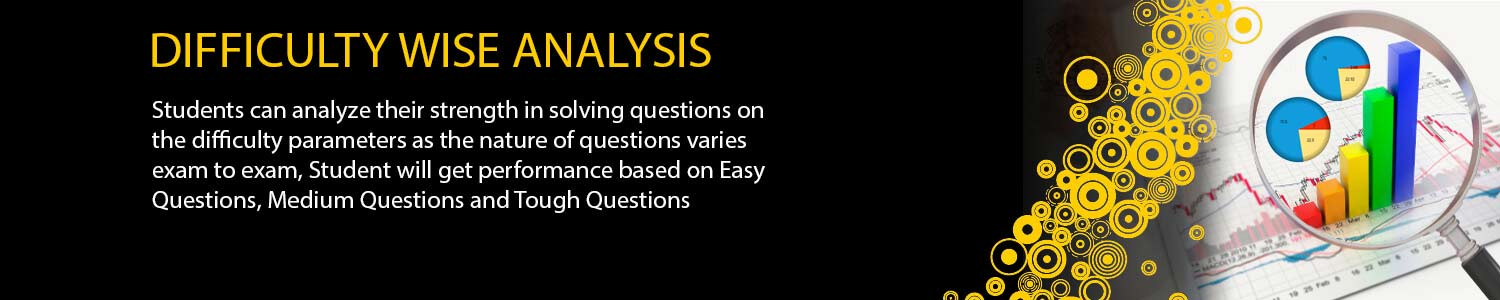## Offers## News & Events# Test Series Schedule

## TEST SERIES SCHEDULE (CSIR-NET/JRF JULY-2020 ONLINE)

# DATE TEST TYPE MODULES Syllabus
1 29-05-2020 MWT-01 Real Analysis I Elementary set theory, finite, countable and uncountable sets, Real number system as a complete ordered field, Archimedean property, supremum, infimum. Sequences and series, convergence, limsup, liminf. Bolzano Weierstrass theorem, Heine Borel theorem. Continuity,monotonic functions, types of discontinuity, uniform continuity, Metric spaces, compactness, connectedness.
2 01-Jun-20 MWT-02 ODE & IE Existence and uniqueness of solutions of initial value problems for first order ordinary differential equations, singular solutions of first order ODEs, system of first order ODEs. General theory of homogenous and non-homogeneous linear ODEs, variation of parameters, Sturm-Liouville boundary value problem. Linear integral equation of the first and second kind of Fredholm and Volterra type, Solutions with separable kernels. Characteristic numbers and eigenfunctions, resolvent kernel.
3 04-Jun-2020 MWT-03 Group Theory & Ring Theory Permutations, combinations, Fundamental theorem of arithmetic, divisibility in Z, congruences, Chinese Remainder Theorem, Euler’s Ø- function, primitive roots. Groups, subgroups, normal subgroups, quotient groups, homomorphisms, cyclic groups, permutation groups, Cayley’s theorem, class equations, Sylow theorems.Rings, ideals, prime and maximal ideals, quotient rings, unique factorization domain, principal ideal domain, Euclidean domain. Polynomial rings and irreducibility criteria. Fields, finite fields, field extensions,
4 07-Jun-2020 MWT-04 Real Analysis II & Functional Analysis differentiability, mean value theorem. Sequences and series of functions, uniform convergence. Riemann sums and Riemann integral, Improper Integrals. functions of bounded variation, Lebesgue measure, Lebesgue integral. Functions of several variables, directional derivative, partial derivative, derivative as a linear transformation, inverse and implicit function theorems. Metric spaces, Topology, compactness, connectedness.
5 10-Jun-2020 MWT-05 Linear Algebra -I Vector spaces, subspaces, linear dependence, basis, dimension, algebra of linear transformations, matrix representation of linear transformation, Algebra of matrices, rank and determinant of matrices, system of linear equations.
6 13-Jun-2020 MWT-06 COV & PDE Variation of a functional, Euler-Lagrange equation, Necessary and sufficient conditions for extrema. Variational methods for boundary value problems in ordinary and partial differential equations. Green’s function. Lagrange and Charpit methods for solving first order PDEs, Cauchy problem for first order PDEs. Classification of second order PDEs, General solution of higher order PDEs with constant coefficients, Method of separation of variables for Laplace, Heat and Wave equations.
7 16-Jun-2020 MWT-07 Linear Algebra-II Eigenvalues and eigenvectors, Cayley-Hamilton theorem. Change of basis, canonical forms, diagonal forms, triangular forms, Jordan forms. Inner product spaces, orthonormal basis. Quadratic forms, reduction and classification of quadratic forms
8 19-Jun-2020 MWT-08 Complex Analysis Algebra of complex numbers, the complex plane, polynomials, power series, transcendental functions such as exponential, trigonometric and hyperbolic functions. Analytic functions, Cauchy-Riemann equations. Contour integral, Cauchy’s theorem, Cauchy’s integral formula, Liouville’s theorem, Maximum modulus principle, Schwarz lemma, Open mapping theorem. Taylor series, Laurent series, calculus of residues. Conformal mappings, Mobius transformations.
9 23-Jun-2020 FLT-01 Full Length Test As per Exam Pattern
10 26-Jun-2020 FLT-02 Full Length Test As per Exam Pattern
11 29-Jun-2020 FLT-03 Full Length Test As per Exam Pattern
12 01-Jul-2020 FLT-04 Full Length Test As per Exam Pattern

## IIT-JAM MATH TEST SERIES 2020

# DATE TEST TYPE MODULES Syllabus
1 3-Jan-20 MWT-01 Group Theory Groups, subgroups, Abelian groups, non-abelian groups, cyclic groups, permutation groups; Normal subgroups, Lagrange's Theorem for finite groups, group homomorphisms and basic concepts of quotient groups (only group theory).
2 6-Jan-20 MWT-02 Integral Calculus Integration as the inverse process of differentiation, definite integrals and their properties, Fundamental theorem of integral calculus. Double and triple integrals, change of order of integration. Calculating surface areas and volumes using double integrals and applications. Calculating volumes using triple integrals and applications.
3 9-Jan-20 MWT-03 Sequences and Series of Real Numbers and LCD Sequences and series of real numbers, Convergent and divergent sequences, bounded and monotone sequences, Convergence criteria for sequences of real numbers, Cauchy sequences, absolute and conditional convergence; Tests of convergence for series of positive terms – comparison test, ratio test, root test; Leibnitz test for convergence of alternating series. limit, continuity, differentiability one variables
4 12-Jan-20 MWT-04 Linear Algebra Vector spaces, Linear dependence of vectors, basis, dimension, linear transformations, matrix representation with respect to an ordered basis, Range space and null space, rank-nullity theorem; Rank and inverse of a matrix, determinant, solutions of systems of linear equations, consistency conditions. Eigenvalues and eigenvectors. Cayley-Hamilton theorem. Symmetric, skew-symmetric, hermitian, skew-hermitian, orthogonal and unitary matrices.
5 15-Jan-20 MWT-05 Differential Equations Ordinary differential equations of the first order of the form y'=f(x,y). Bernoulli’s equation, exact differential equations, integrating factor, Orthogonal trajectories, Homogeneous differential equations-separable solutions, Linear differential equations of second and higher order with constant coefficients, method of variation of parameters. Cauchy-Euler equation.
6 18-Jan-20 MWT-06 Real Analysis: 1 Interior points, limit points, open sets, closed sets, bounded sets, connected sets, compact sets; completeness of R, Power series (of real variable) including Taylor’s and Maclaurin’s, domain of convergence, term-wise differentiation and integration of power series.
7 21-Jan-20 MWT-07 Vector calculus Scalar and vector fields, gradient, divergence, curl and Laplacian. Scalar line integrals and vector line integrals, scalar surface integrals and vector surface integrals, Green's, Stokes and Gauss theorems and their applications.
8 24-Jan-20 MWT-08 Real Analysis: 2 limit, continuity, partial derivatives, differentiability in two Variables , inccreasing and decreasing , maxima and minima one variable and two variable , Chain Rule, Inverse function theorem
9 27-Jan-20 FLT-01 Full Length Test As per Exam Pattern
10 30-Jan-20 FLT-02 Full Length Test As per Exam Pattern
11 2-Feb-20 FLT-03 Full Length Test As per Exam Pattern
12 5-Feb-20 FLT-04 Full Length Test As per Exam Pattern

## IIT-JAM STATS TEST SERIES 2020

# DATE TEST TYPE MODULES Syllabus
1 28 Dec 19 MWT-01 Basic Probability and Random Variables Probability: Axiomatic definition of probability and properties, conditional probability, multiplication rule. Theorem of total probability. Bayes' theorem and independence of events.Random Variables: Probability mass function, probability density function and cumulative distribution functions, distribution of a function of a random variable. Mathematical expectation, moments and moment generating function.
2 1 Jan 20 MWT-02 Standard Distribution Standard Distributions: Binomial, negative binomial, geometric, Poisson, hypergeometric, uniform, exponential, gamma, beta and normal distributions. Poisson and normal approximations of a binomial distribution. Sampling distributions: Chi-square, t and F distributions, and their properties.
3 5 Jan 20 MWT-03 Joint Distribution & Limit Theorem Joint Distributions: Joint, marginal and conditional distributions. Distribution of functions of random variables. Joint moment generating function. Product moments, correlation, simple linear regression. Independence of random variables.Limit Theorems: Weak law of large numbers. Central limit theorem (i.i.d.with finite variance case only).Chebyshev's inequality.
4 9 Jan 20 MWT-04 Estimation Unbiasedness, consistency and efficiency of estimators, method of moments and method of maximum likelihood. Sufficiency, factorization theorem. Completeness, Rao-Blackwell and Lehmann-Scheffe theorems, uniformly minimum variance unbiased estimators. Rao-Cramer inequality. Confidence intervals for the parameters of univariate normal, two independent normal, and one parameter exponential distributions.
5 13 Jan 20 MWT-05 Testing of Hytothesis Basic concepts, applications of Neyman-Pearson Lemma for testing simple and composite hypotheses. Likelihood ratio tests for parameters of univariate normal distribution. Basic concepts, applications of Neyman-Pearson Lemma for testing simple and composite hypotheses. Likelihood ratio tests for parameters of univariate normal distribution.
6 16 Jan 20 MWT-06 Real Analysis Sequences and Series: Convergence of sequences of real numbers, Comparison, root and ratio tests for convergence of series of real numbers. Differential Calculus: Limits, continuity and differentiability of functions of one and two variables. Rolle's theorem, mean value theorems, Taylor's theorem, indeterminate forms, maxima and minima of functions of one and two variables.
7 20 Jan 20 MWT-07 Linear Algebra Matrices: Rank, inverse of a matrix. Systems of linear equations. Linear transformations, eigenvalues and eigenvectors. Cayley-Hamilton theorem, symmetric, skew-symmetric and orthogonal matrices.
8 22 Jan 20 MWT-08 Integral Calculus Integral Calculus: Fundamental theorems of integral calculus. Double and triple integrals, applications of definite integrals, arc lengths, areas and volumes.
9 25 Jan 20 FLT-01 Full Length Test As per Exam Pattern.
10 28 Jan 20 FLT-02 Full Length Test As per Exam Pattern.
11 30 Jan 20 FLT-03 Full Length Test As per Exam Pattern.
12 1 Feb 20 FLT-04 Full Length Test As per Exam Pattern.

## Offers## News & Events